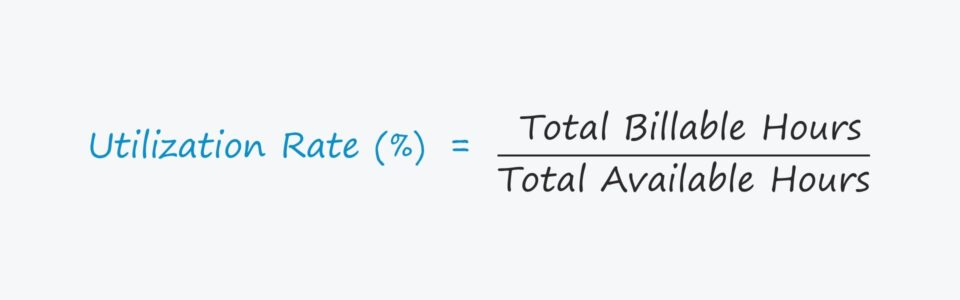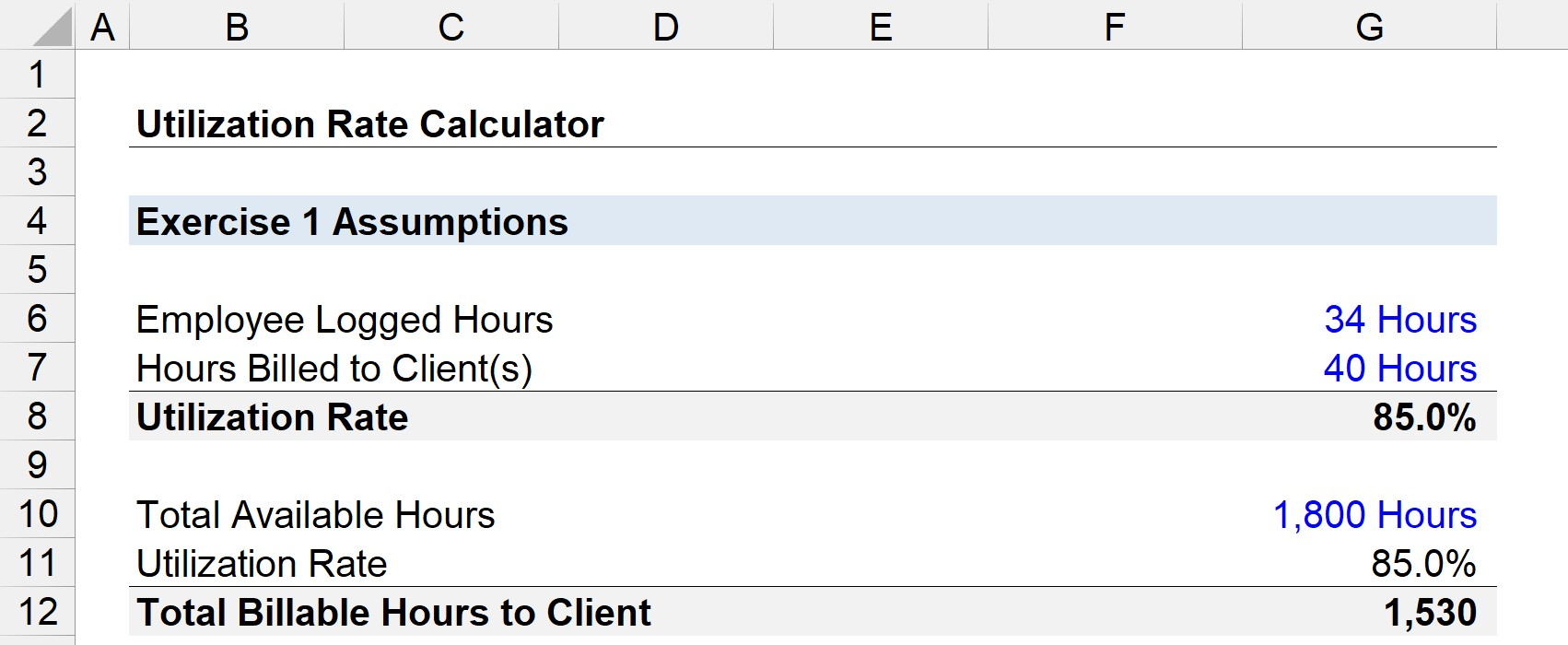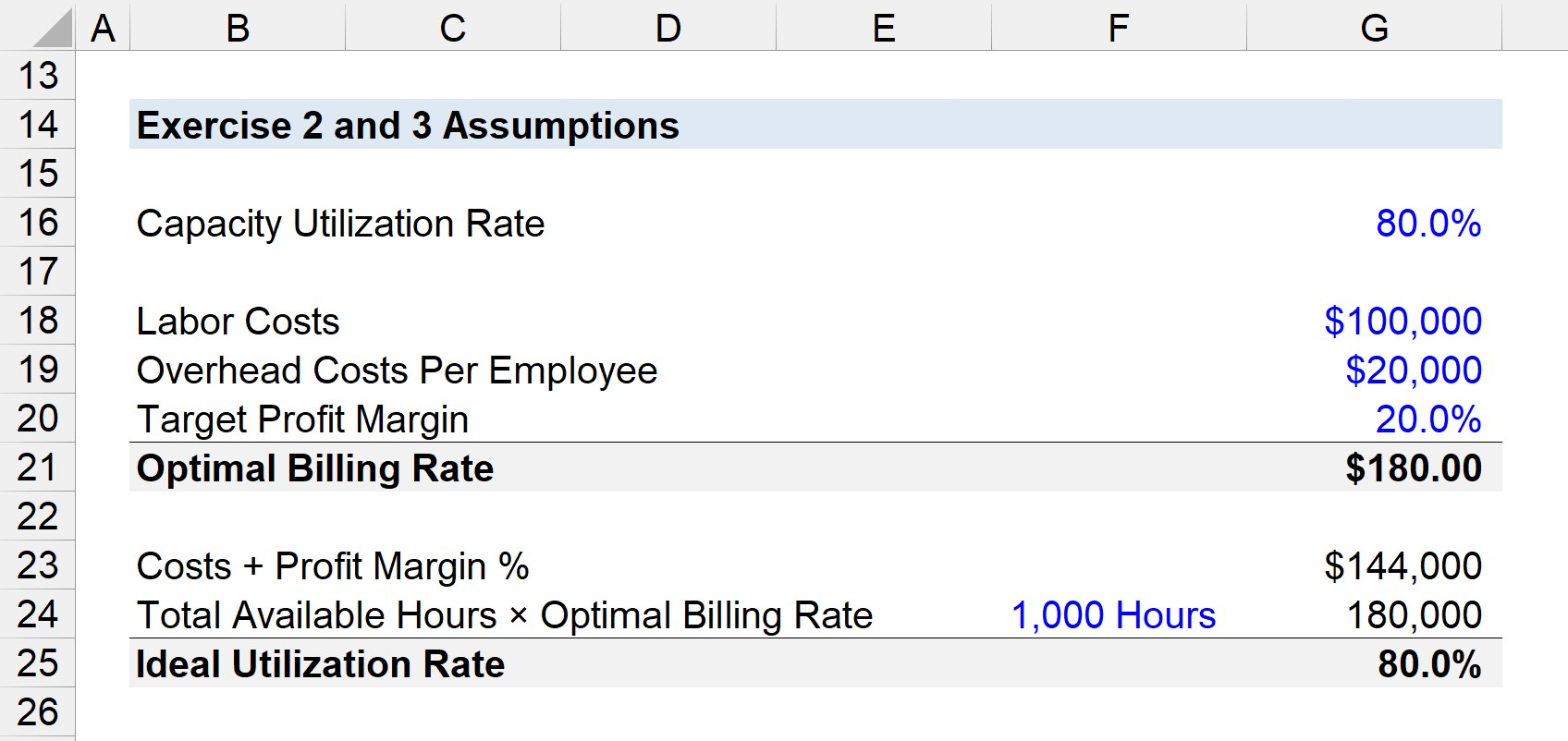Welcome to Wall Street Prep! Use code at checkout for 15% off.# Utilization Rate

Guide to Understanding the Utilization Rate## How to Calculate Utilization Rate?

The utilization rate is defined as the percentage of an employee’s total working hours spent productively, i.e. the hours billable to a client.

Conceptually, the utilization rate measures the percentage of an employee’s total working hours spent on productive work for clients.

Utilization is the amount of an employee’s total available time — i.e. work capacity — used for productive work that is billable to clients, expressed as a percentage.

Time is a constraint, so ensuring each hour is spent efficiently with limited waste is crucial for productivity.

In particular, companies with business models oriented around billing clients by the hour — e.g. consulting firms, law firms, and marketing agencies — must confirm their hourly rate sufficiently covers all their expenses to be profitable.

## Utilization Rate Formula

Calculating the utilization rate consists of dividing an employee’s total billable hours by the total available hours.

Utilization Rate = Total Billable Hours ÷ Total Available Hours

In order to express the rate in percentage form, the resulting figure should be multiplied by 100.

With the insights derived from the metric, a company’s management team can set pricing, hire new employees, and offer salaries where profit margins are maximized.

## What is a Good Utilization Rate?

For the most part, higher utilization is preferable, as it means more hours are being spent in a time-efficient manner. Yet, if a company’s utilization is consistently near or even at 100%, that implies the employees could be overworked and are close to burnout.

While spending too much time on non-billable hours and unproductive tasks signals a need for improved operational measures, there must be a balance between remaining on task most of the time and ensuring high employee morale.

Otherwise, even if the employees are technically “efficient,” their work quality will start to show signs of deterioration, which will inevitably be noticed by clients.

The utilization varies by role as well as position (i.e. rank in the organization hierarchy).

Top executives and upper-level employees generally have lower utilization — which does not mean that they are less efficient, but that more of their time is allocated towards winning client work, managing employees, internal planning, delegating work, etc. For instance, eating dinner with a client to pitch their team’s services does not count as billable work, but is how the project pipeline is built to obtain client work later.

Further down the hierarchy structure, “front-line” employees are expected to have higher utilization since their responsibility is client-facing (i.e. directly working with clients).

## Utilization Rate Calculator

We’ll now move to a modeling exercise, which you can access by filling out the form below.Submitting...

## 1. Employee Utilization Rate Calculation Example

Suppose an employee is paid on the expectation of logging 40 hours of work per week.

If that employee billed clients for 34 of those hours, the utilization for the week is 85%.

• Utilization Rate = 34 Hours ÷ 40 Hours = .85, or 85%

Therefore, if that employee were to hypothetically work 1,800 hours (i.e. the total available hours), the number of billable hours to clients would be estimated at 1,530.

• Total Billable Hours = 1,800 Hours × 85% = 1,530## 2. Capacity Utilization Rate Formula

The capacity utilization rate is the utilization for a company’s average employee, making it more encompassing as all employees are accounted for rather than just one individual.

The formula for the capacity utilization rate consists of dividing all employee utilization rates by the total number of employees.

Capacity Utilization Rate = Total Employee Utilization Rates ÷ Total Number of Employees

While the utilization rate can be used to identify underperforming employees and operational weaknesses, the success of an enterprise is largely contingent on capacity utilization — albeit the two are closely interconnected.

More specifically, the efficiency of one employee cannot offset the inefficient, unproductive work of others, particularly in larger companies.

Plus, ineffective team workload management where there is significant reliance on only a handful of employees to produce most of the output is frequently the cause of an employee burning themselves out.

## 3. Optimal Billing Rate Formula

Once a company’s utilization has been calculated, the next step is to determine how much to charge clients (i.e. the hourly rate) in order to meet its profit margin targets, i.e. the optimal billing rate.

The optimal billing rate is the hourly rate that an enterprise needs to charge to turn a profit based on the average employee utilization.

Optimal Billing Rate = [(Labor Costs + Overhead Costs + Profit Margin) ÷ (Total Labor Hours)] ÷ Capacity Utilization Rate

Suppose a company’s total labor costs are \$100,000, there are \$20,000 in overhead costs per employee, and the target profit margin is 20%.

• Labor Costs = \$100,000
• Overhead Costs Per Employee = \$20,000
• Target Profit Margin = 20%

Note how the numerator must be adjusted, i.e. the sum (\$144,000) must be divided by the total average labor hours (1,000).

If the total labor hours are 1,000, then the numerator equals 144

• [\$100,000 + \$20,000 + (20% × \$120,000)] ÷ 1,000 = 144

Then, assuming a capacity utilization of 80%, the optimal billing rate comes out to \$180.00 per hour.

• Optimal Billing Rate = 144 ÷ 80% = \$180.00

## 4. Ideal Utilization Rate Formula

The ideal utilization rate can be derived using a target billing rate — which is set based on the average employee utilization and optimal billing rate, among other factors — where its target profit margin is met.

The ideal utilization formula divides the sum of its resource costs, overhead costs, and profit margin by the total available hours multiplied by the optimal billing rate.

Ideal Utilization Rate = (Resource Costs + Overhead Costs + Profit Margin) ÷ (Total Available Hours × Optimal Billing Rate)

Given the same assumptions as in the previous example, the ideal utilization rate is 80%.

• Ideal Utilization Rate = \$144,000 ÷ (1,000 × 80%) = 80%

The 80% represents an enterprise’s optimal utilization to meet its target profit margin, which would then be compared to its capacity utilization to determine if any operational improvements are necessary.Step-by-Step Online Course

### Everything You Need To Master Financial Modeling

Enroll in The Premium Package: Learn Financial Statement Modeling, DCF, M&A, LBO and Comps. The same training program used at top investment banks.Inline FeedbacksLearn Financial Modeling Online

Everything you need to master financial and valuation modeling: 3-Statement Modeling, DCF, Comps, M&A and LBO.

X

The Wall Street Prep Quicklesson Series

7 Free Financial Modeling Lessons

Get instant access to video lessons taught by experienced investment bankers. Learn financial statement modeling, DCF, M&A, LBO, Comps and Excel shortcuts.